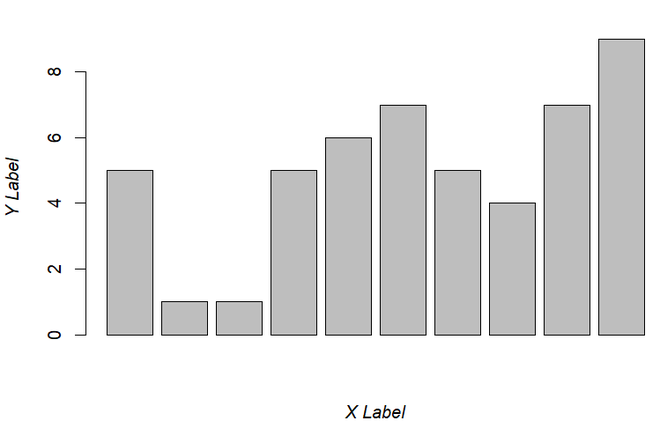GFG App
Open AppBrowser
Continue

In this article, we are going to see how to use italic font using various functionalities in the R programming language.

## Italic font using substitute(), paste() and italic() functions

In this approach to use the italic function, the user needs to use three different functions within each other, and the italic font will be applied to the given text, starting with the substitute() function; within this function, the user needs to call the paste function as the argument of the substitute function, further within the paste function the user needs to call the italic() function as the argument of the paste function and then in the italic function the user has to pass the text which is needed to be in the italic font in the R programming language.

### Syntax to produce italic font in R:

substitute(paste(italic(‘text’)))

• Substitute() function: This function will return the parse tree for the (unevaluated) expression expr, substituting any variables bound in env.

Syntax: substitute(expr, env)

Parameters:

• expr: any syntactically valid R expression
• env: an environment or a list object. Defaults to the current evaluation environment.
• Paste() function: This function is used to concatenate vectors after converting them to characters.

Syntax: paste (…, sep = ” “, collapse = NULL)

Parameters:

• …: one or more R objects, to be converted to character vectors.
• sep: a character string to separate the terms. Not NA_character_.
• collapse: an optional character string to separate the results. Not NA_character_.
• Italic function: This function is used to change the font decoration of selected rows and columns of a flexible.

Syntax: italic(x, i = NULL, j = NULL, italic = TRUE, part = “body”)

Parameters:

• x: a flextable object
• I: rows selection
• j: columns selection
• italic: boolean value
• part: partname of the table (one of ‘all’, ‘body’, ‘header’, ‘footer’)

## Example 1:Italic Font in Title of Plot

In this example, we will be using all three functions to apply the italic font at the main of the bar plot given in the R programming language.

## R

 `a = ``c``(5,1,1,5,6,7,5,4,7,9)` `barplot``(a,main = ``substitute``(` `  ``paste``(``italic``(` `    ``'GeeksforGeeks is the best learning\` `platform ``for` `CSE Graduates.'))))`

Output:## Example 2: Italic Font on Axis Labels of Plot

In this example, we will be using all three functions to apply the italic font at the given bar plot at the x-axis and the y-axis of the barplot in the R programming language.

## R

 `a = ``c``(5,1,1,5,6,7,5,4,7,9)` `barplot``(a, xlab = ``substitute``(``paste``(``italic``(``'X Label'``))),` `               ``ylab = ``substitute``(``paste``(``italic``(``'Y Label'``))))`

Output:## Example 3: Italic Font with Text

In this example, we will be using all three functions to apply the italic font at the given bar plot in the R programming language.

## R

 `a = ``c``(5,1,1,5,6,7,5,4,7,9)` `barplot``(a)` `text``(6, 8, ``substitute``(``paste``(` `  ``italic``('GeeksforGeeks is the best learning\` `platform ``for` `CSE Graduates.'))))`

Output:My Personal Notes arrow_drop_up